# Rigged Hilbert space

(diff) ← Older revision | Latest revision (diff) | Newer revision → (diff)

A Hilbert spacecontaining a linear, everywhere-dense subset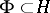, on which the structure of a topological vector space is defined, such that the imbedding is continuous. This imbedding generates a continuous imbedding of the dual space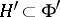and a chain of continuous imbeddings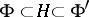(using the standard identification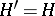). The most interesting case is that in whichis a nuclear space. The following strengthening of the spectral theorem for self-adjoint operators acting onis true: Any self-adjoint operatormappingcontinuously (in the topology of) onto itself possesses a complete system of generalized eigenfunctions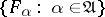(is a set of indices), i.e. elements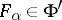such that for any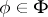,where the set of values of the function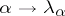,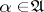, is contained in the spectrum of(cf. Spectrum of an operator) and has full measure with respect to the spectral measure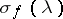,,, of any element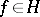. The completeness of the system means that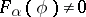for any,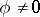, for at least one. Moreover, for any element, its expansion with respect to the system of generalized eigenfunctionsexists and generalizes the known expansion with respect to the basis of eigenvectors for an operator with a discrete spectrum.

Example: The expansion into a Fourier integral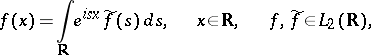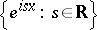is a system of generalized eigenfunctions of the differentiation operator, acting on, arising under the natural rigging of this space by the Schwartz space(cf. Generalized functions, space of). The same assertions are also correct for unitary operators acting on a rigged Hilbert space.

How to Cite This Entry:
Rigged Hilbert space. Encyclopedia of Mathematics. URL: http://encyclopediaofmath.org/index.php?title=Rigged_Hilbert_space&oldid=13083
This article was adapted from an original article by R.A. Minlos (originator), which appeared in Encyclopedia of Mathematics - ISBN 1402006098. See original article